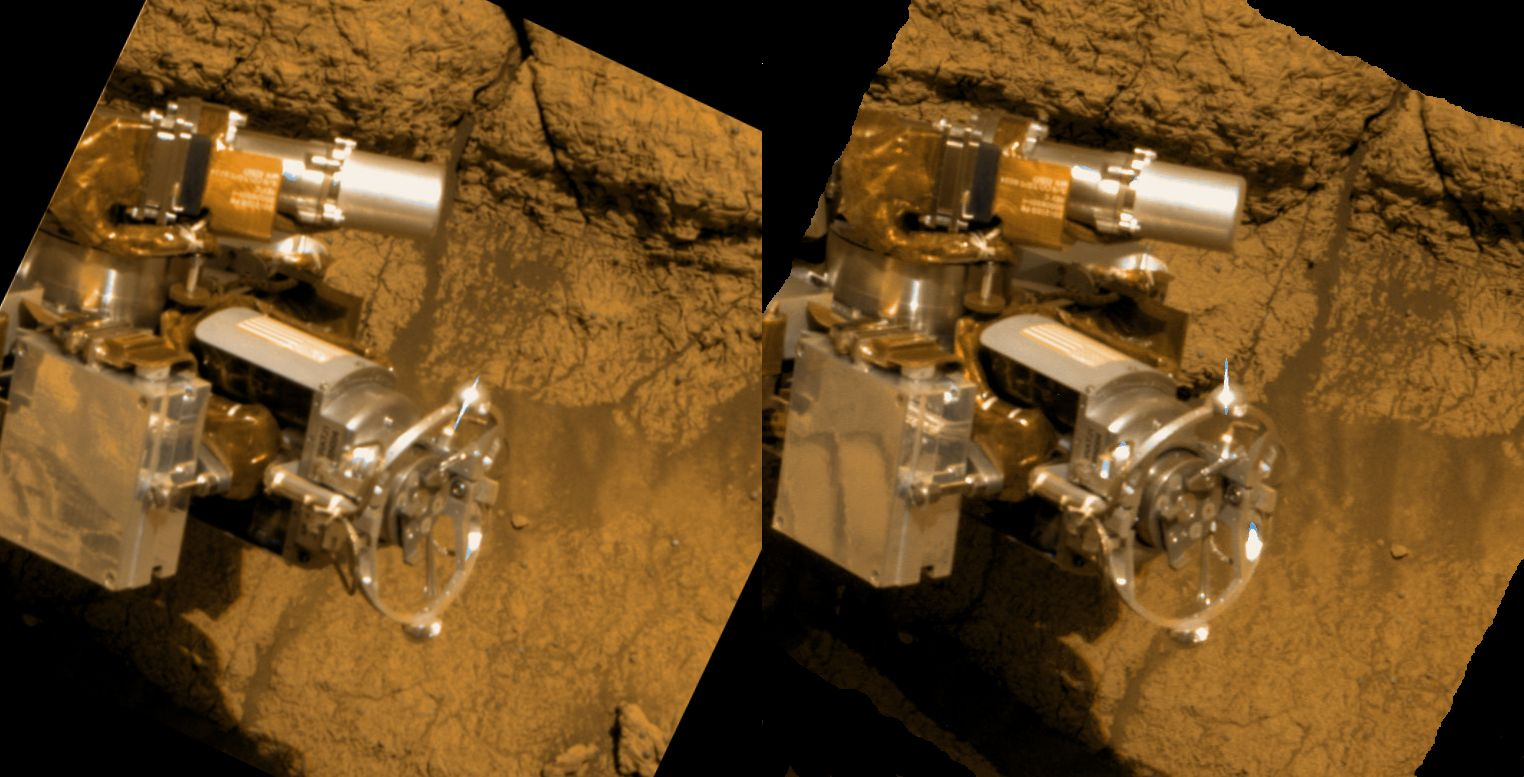Here’s a little undocumented function to horizontally concatenate two `cv::Mat` matrices (with the same number of rows).It is used like this:

`````` cv::Mat m1(10, 5, CV_32FC3);
cv::Mat m2(10, 3, CV_32FC3);
cv::Mat m3;
cv::hconcat(m1, m2, m3);
assert(10 == m3.rows && 8 == m3.cols);

cv::hconcat(std::vector<cv::Mat>{m1, m2}, m3);
assert(10 == m3.rows && 8 == m3.cols);

cv::Mat matArray[] = { m1, m2 };
cv::hconcat(matArray, 2, m3); // Accept array + size
assert(10 == m3.rows && 8 == m3.cols);
``````

The function `cv::hconcat()` has 2 additional overloads: One for accepting an array of `cv::Mat`s and array size, and another for accepting a `cv::InputArrayOfArrays` which is essentially an `std::vector<cv::Mat>`.

Note that this function will copy all the elements of input matrices and (possibly) reallocate the output matrix.

• Header declaration in OpenCV: core.hpp;
• Hat tip to SO answer.
• The image from here shows a section of rock that has being ground away by the Rock Abrasion Tool (RAT) of the Opportunity rover on Mars.